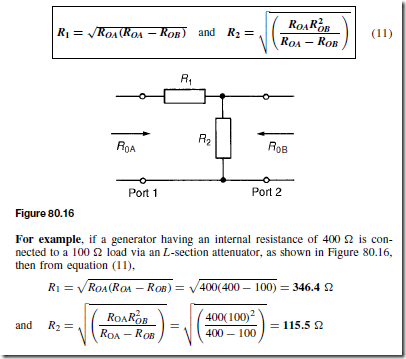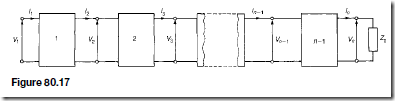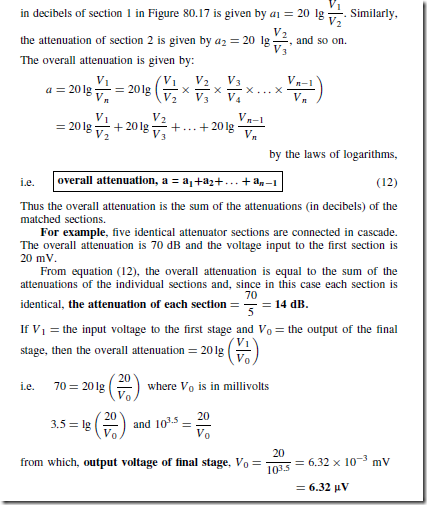### Newton's third law of motion ( Action and Reaction ! )

When a bullet is fired from a gun equal and opposite forces are exerted on the bullet and the gun during the time the bullet is passing down the barrel

### Newton's second law of motion ( momentum , force , weight , free falling )

A motortruck requires a larger force to set in motion when it is heavily loaded than when it is empty

### The nature of friction: Static and dynamic friction

Friction is the name given to the force which opposes the relative sliding motion of two surfaces in contact with one another. It plays a notable part in our daily lives. For example

### Newton's Laws of motion:1- Newton's first law of motion

In 1687 Newton published a book written , as was the routine in those days , in Latin and given the title , Philosophiae naturalis principia mathematica Translated , this means

### Derivation of Kinetic energy formula and worked examples

Where there are no opposing forces, a moving body needs noforce to keep it moving with a steady velocity ( Newton's first law of motion ). If, however, a resultant force does act on a moving body

# Transmission Lines

### Introduction

A transmission line is a system of conductors connecting one point to another and along which electromagnetic energy can be sent. Thus telephone lines and power distribution lines are typical examples of transmission lines; in electronics, however, the term usually implies a line used for the transmission of radio-frequency (r.f.) energy such as that from a radio transmitter to the antenna.

An important feature of a transmission line is that it should guide energy from a source at the sending end to a load at the receiving end without loss by radiation. One form of construction often used consists of two similar conductors mounted close together at a constant separation. The two conductors form the two sides of a balanced circuit and any radiation from one of them is neutralised by that from the other. Such twin-wire lines are used for carrying high r.f. power, for example, at transmitters. The coaxial form of construction is commonly employed for low power use, one conductor being in the form of a cylinder that surrounds the other at its centre, and thus acts as a screen. Such cables are often used to couple f.m. and television receivers to their antennas.

At frequencies greater than 1000 MHz, transmission lines are usually in the form of a waveguide, which may be regarded as coaxial lines without the centre conductor, the energy being launched into the guide or abstracted from it by probes or loops projecting into the guide.

#### Transmission Line Primary Constants

Let an a.c. generator be connected to the input terminals of a pair of parallel conductors of infinite length. A sinusoidal wave will move along the line and a finite current will flow into the line. The variation of voltage with distance along the line will resemble the variation of applied voltage with time. The moving wave, sinusoidal in this case, is called a voltage travelling wave. As the wave moves along the line the capacitance of the line is charged up and the moving charges cause magnetic energy to be stored. Thus the propagation of such an electromagnetic wave constitutes a flow of energy.

After sufficient time the magnitude of the wave may be measured at any point along the line. The line does not therefore appear to the generator as an open circuit but presents a definite load Z0. If the sending-end voltage is VS and the sending end current is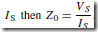. Thus, the line absorbs all of the energy and the line behaves in a similar manner to the generator, as would a single ‘lumped’ impedance of value Z0 connected directly across the generator terminals.

There are four parameters associated with transmission lines, these being resistance, inductance, capacitance and conductance.

(i) Resistance R is given by R + pl/A, where p is the resistivity of the conductor material, A is the cross-sectional area of each conductor and l is the length of the conductor (for a two-wire system, l represents twice the length of the line). Resistance is stated in ohms per metre length of a line and represents the imperfection of the conductor. A resistance stated in ohms per loop metre is a little more specific since it takes into consideration the fact that there are two conductors in a particular length of line.

(ii) Inductance L is due to the magnetic field surrounding the conductors of a transmission line when a current flows through them. The inductance

of an isolated twin line is considered in chapter 79. From equation (11),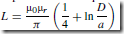henry/ metre, where D is the distance between centres of the conductor and a is the radius of each conductor. In most practical lines µr D 1. An inductance stated in henrys per loop metre takes into consideration the fact that there are two conductors in a particular length of line.

(iii) Capacitance C exists as a result of the electric field between conductors of a transmission line. The capacitance of an isolated twin line is considered in chapter 79. From equation (7), page 594, the capacitance between the two conductors is given by: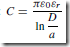farads/metre. In most practical lines εr D 1.

(iv) Conductance G is due to the insulation of the line allowing some current to leak from one conductor to the other. Conductance is measured in siemens per metre length of line and represents the imperfection of the insulation. Another name for conductance is leakance.

Each of the four transmission line constants, R, L, C and G, known as the primary constants, are uniformly distributed along the line.

From chapter 80, when a symmetrical T-network is terminated in its characteristic impedance Z0, the input impedance of the network is also equal to Z0. Similarly, if a number of identical T-sections are connected in cascade, the input impedance of the network will also be equal to Z0.

A transmission line can be considered to consist of a network of a very large number of cascaded T-sections each a very short length (υl) of transmission line, as shown in Figure 83.1. This is an approximation of the uniformly distributed line; the larger the number of lumped parameter sections, the nearer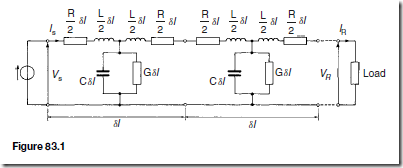it approaches the true distributed nature of the line. When the generator VS is connected, a current IS flows which divides between that flowing through the leakage conductance G, which is lost, and that which progressively charges each capacitor C and which sets up the voltage travelling wave moving along the transmission line. The loss or attenuation in the line is caused by both the conductance G and the series resistance R.

#### Phase Delay, Wavelength and Velocity of Propagation

Each section of that shown in Figure 83.1 is simply a low-pass filter possessing losses R and G. If losses are neglected, and R and G are removed, the circuit simplifies and the infinite line reduces to a repetitive T-section low-pass filter network as shown in Figure 83.2. Let a generator be connected to the line as shown and let the voltage be rising to a maximum positive value just at the instant when the line is connected to it. A current IS flows through inductance L1 into capacitor C1. The capacitor charges and a voltage develops across it. The voltage sends a current through inductance L0 and L2 into capacitor

C2. The capacitor charges and the voltage developed across it sends a current through L0 and L3 into C3, and so on. Thus all capacitors will in turn charge up to the maximum input voltage. When the generator voltage falls, each capacitor is charged in turn in opposite polarity, and as before the input charge is progressively passed along to the next capacitor. In this manner voltage and current waves travel along the line together and depend on each other.

The process outlined above takes time; for example, by the time capacitor C3 has reached its maximum voltage, the generator input may be at zero or moving towards its minimum value. There will therefore be a time, and thus a phase difference between the generator input voltage and the voltage at any point on the line.

##### Phase delay

Since the line shown in Figure 83.2 is a ladder network of low-pass T-section filters, it may be shown that the phase delay, ˇ, is given by: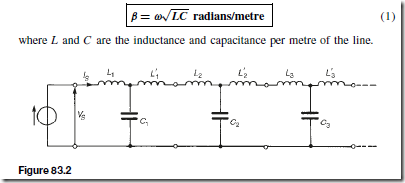Wavelength

The wavelength A on a line is the distance between a given point and the next point along the line at which the voltage is the same phase, the initial point leading the latter point by 2ˇ radian. Since in one wavelength a phase change of 2ˇ radians occurs, the phase change per metre is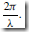Hence, phase change per metre,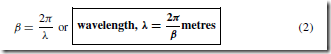Velocity of propagation

The velocity of propagation, u, is given by u D fA, where f is the frequency and A the wavelength. Hence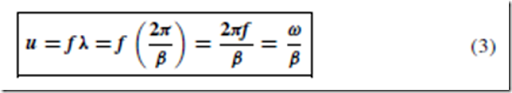The velocity of propagation of free space is the same as that of light, i.e. approximately 300 x 106 m/s. The velocity of electrical energy along a line is always less than the velocity in free space. The wavelength A of radiation in free space is given by A + f where c is the velocity of light. Since the velocity along a line is always less than c, the wavelength corresponding to any particular frequency is always shorter on the line than it would be in free space.

For example, a transmission line has an inductance of 4 mH/loop km and a capacitance of 0.004 µF/km. For a frequency of operation of 1 kHz, from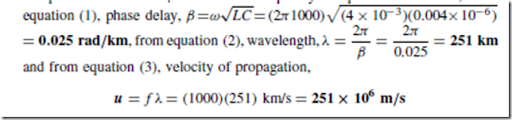Current and Voltage Relationships

Figure 83.3 shows a voltage source VS applied to the input terminals of an infinite line, or a line terminated in its characteristic impedance, such that a current IS flows into the line. At a point, say, 1 km down the line let the current be I1 . The current I1 will not have the same magnitude as IS because of line attenuation; also I1 will lag IS by some angle ˇ. The ratio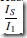is therefore a phasor quantity. Let the current a further 1km down the line be I2 , and so on, as shown in Figure 83.3. Each unit length of line can be treated as a section of a repetitive network, and the attenuation is in the form of a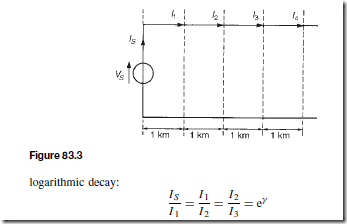where y is the propagation constant. y has no unit.

The propagation constant is a complex quantity given by y = a+jB where ˛ is the attenuation constant, whose unit is the neper, and ˇ is the phase shift coefficient, whose unit is the radian. For n such 1 km sections,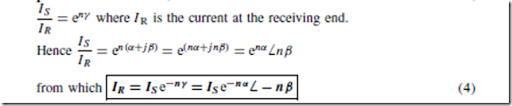In equation (4), the attenuation on the line is given by n˛ nepers and the phase shift is nˇ radians.

At all points along an infinite line, the ratio of voltage to current is Z0, the characteristic impedance. Thus from equation (4) it follows that: receiving end voltage,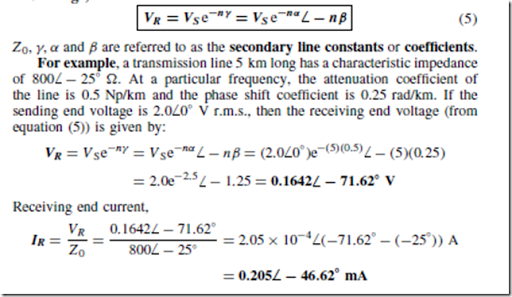Characteristic Impedance and Propagation Coefficient in Terms of the Primary Line Constants

At all points along an infinite line, the ratio of voltage to current is called the characteristic impedance Z0. The value of Z0 is independent of the length of the line; it merely describes a property of a line that is a function of the physical construction of the line. Since a short length of line may be considered as a ladder of identical low-pass filter sections, the characteristic impedance may be determined from chapter 80, i.e.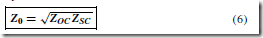since the open-circuit impedance ZOC and the short-circuit impedance ZSC may be easily measured.

The characteristic impedance of a transmission line may also be expressed in terms of the primary constants, R, L, G and C. Measurements of the primary constants may be obtained for a particular line and manufacturers usually state them for a standard length.

It may be shown that the characteristic impedance Z0 is given by: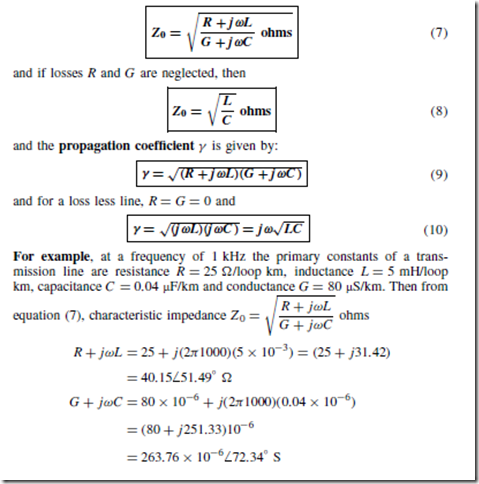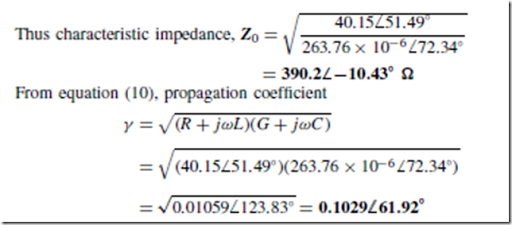Distortion on Transmission Lines

If the waveform at the receiving end of a transmission line is not the same shape as the waveform at the sending end, distortion is said to have occurred.

In designing a transmission line, if LG = CR no distortion is introduced. This means that the signal at the receiving end is the same as the sending-end signal except that it is reduced in amplitude and delayed by a fixed time. Also, with no distortion, the attenuation on the line is a minimum.

In practice, however, The inductance is usually low and the capacitance is large and not easily reduced. Thus if the condition LG D CR is to be achieved in practice, either L or G must be increased since neither C or R can really be altered. It is undesirable to increase G since the attenuation and power losses increase. Thus the inductance L is the quantity that needs to be increased and such an artificial increase in the line inductance is called loading. This is achieved either by inserting inductance coils at intervals along the transmission line — this being called ‘lumped loading’ — or by wrapping the conductors with a high-permeability metal tape — this being called ‘continuous loading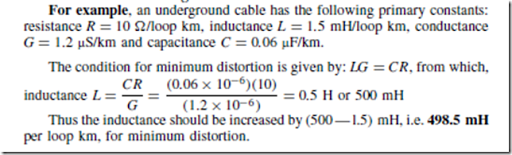Wave Reflection and Reflection Coefficient

In earlier sections of this chapter it was assumed that the transmission line had been properly terminated in its characteristic impedance or regarded as an infinite line. In practice, of course, all lines have a definite length and often the terminating impedance does not have the same value as the characteristic

impedance of the line. When this is the case, the transmission line is said to have a ‘mismatched load’.

The forward-travelling wave moving from the source to the load is called the incident wave or the sending-end wave. With a mismatched load the termination will absorb only a part of the energy of the incident wave, the remainder being forced to return back along the line toward the source. This latter wave is called the reflected wave.

A transmission line transmits electrical energy; when such energy arrives at a termination that has a value different from the characteristic impedance, it experiences a sudden change in the impedance of the medium. When this occurs, some reflection of incident energy occurs and the reflected energy is lost to the receiving load. Reflections commonly occur in nature when a change of transmission medium occurs; for example, sound waves are reflected at a wall, which can produce echoes (see Chapter 17), and mirrors reflect light rays (see Chapter 19).

If a transmission line is terminated in its characteristic impedance, no reflection occurs; if terminated in an open circuit or a short circuit, total reflection occurs, i.e. the whole of the incident wave reflects along the line. Between these extreme possibilities, all degrees of reflection are possible.

##### Energy associated with a travelling wave

A travelling wave on a transmission line may be thought of as being made up of electric and magnetic components. Energy is stored in the magnetic field due to the current (energy D 1 LI2 — see page 597) and energy is stored in the electric field due to the voltage (energy D 1 CV2 — see page 594). It is the continual interchange of energy between the magnetic and electric fields, and vice versa, that causes the transmission of the total electromagnetic energy along the transmission line.

When a wave reaches an open-circuited termination the magnetic field collapses since the current I is zero. Energy cannot be lost, but it can change form. In this case it is converted into electrical energy, adding to that already caused by the existing electric field. The voltage at the termination consequently doubles and this increased voltage starts the movement of a reflected wave back along the line. This movement will set up a magnetic field and the total energy of the reflected wave will again be shared between the magnetic and electric field components.

When a wave meets a short-circuited termination, the electric field col- lapses and its energy changes form to the magnetic energy. This results in a doubling of the current.

##### Reflection coefficient

The ratio of the reflected current to the incident current is called the reflection coefficient and is often given the symbol p, i.e.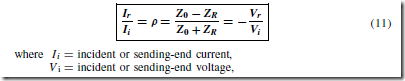For example, a cable, which has a characteristic impedance of 75 ω, is terminated in a 250 ω resistive load. Assume that the cable has negligible losses and the voltage measured across the terminating load is 10 V.

From equation (11), reflection coefficient,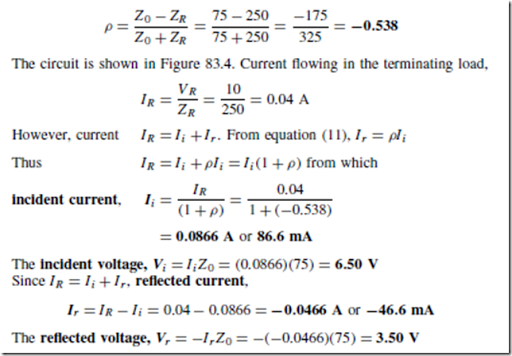Standing Waves and Standing Wave Ratio

Consider a loss free transmission line open-circuited at its termination. An incident current waveform is completely reflected at the termination, and, as stated in earlier, the reflected current is of the same magnitude as the incident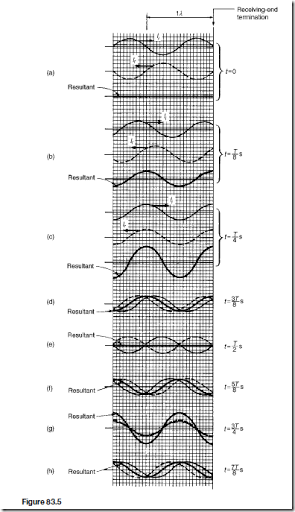current but is 180° out of phase. Figure 83.5(a) shows the incident and reflected current waveforms drawn separately (shown as Ii moving to the right and Ir moving to the left respectively) at a time t D 0, with Ii D 0 and decreasing at the termination.

The resultant of the two waves is obtained by adding them at intervals. In this case the resultant is seen to be zero. Figures 83.5(b) and (c) show the incident and reflected waves drawn separately as times t D T/8 seconds and t D T/4, where T is the periodic time of the signal. Again, the resul- tant is obtained by adding the incident and reflected waveforms at intervals. Figures 83.5(d) to (h) show the incident and reflected current waveforms plot- ted on the same axis, together with their resultant waveform, at times t D 3T/8 to t D 7T/8 at intervals of T/8.

If the resultant waveforms shown in Figures 83.5(a) to (g) are superimposed one upon the other, Figure 83.6 results. (Note that the scale has been doubled for clarity.) The waveforms show clearly that waveform (a) moves to (b) after T/8, then to (c) after a further period of T/8, then to (d), (e), (f), (g) and (h) at intervals of T/8. It is noted that at any particular point the cur- rent varies sinusoidally with time, but the amplitude of oscillation is different at different points on the line.

Whenever two waves of the same frequency and amplitude travelling in opposite directions are superimposed on each other as above, interference takes place between the two waves and a standing or stationary wave is produced. The points at which the current is always zero are called nodes (labelled N in Figure 83.6). The standing wave does not progress to the left or right and the nodes do not oscillate. Those points on the wave that undergo maximum distur- bance are called antinodes (labelled A in Figure 83.6). The distance between adjacent nodes or adjacent antinodes is A/2, where A is the wavelength. A standing wave is therefore seen to be a periodic variation in the vertical plane taking place on the transmission line without travel in either direction The resultant of the incident and reflected voltage for the open-circuit termination may be deduced in a similar manner to that for current. However, as stated earlier, when the incident voltage wave reaches the termination it is reflected without phase change. Figure 83.7 shows the resultant waveforms of incident and reflected voltages at intervals of t D T/8. Figure 83.8 shows all the resultant waveforms of Figure 83.7(a) to (h) superimposed; again, standing waves are seen to result. Nodes (labelled N) and antinodes (labelled A) are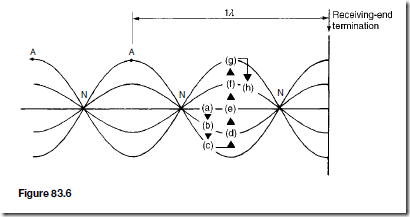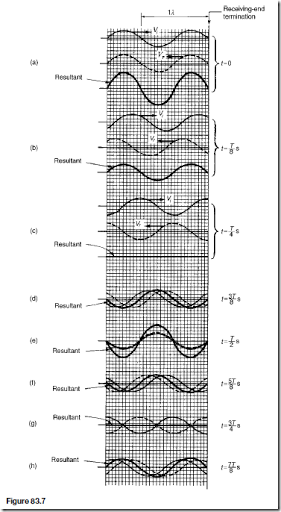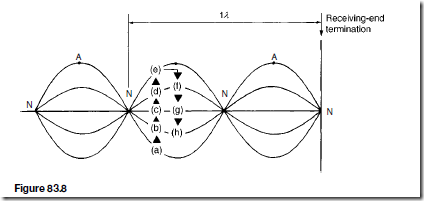shown in Figure 83.8 and, in comparison with the current waves, are seen to occur 90° out of phase.

If the transmission line is short-circuited at the termination, it is the incident current that is reflected without phase change and the incident voltage that is reflected with a phase change of 180° . Thus the diagrams shown in Figures 83.5 and 83.6 representing current at an open-circuited termination may be used to represent voltage conditions at a short-circuited termination and the diagrams shown in Figures 83.7 and 83.8 representing voltage at an open-circuited termination may be used to represent current conditions at a short-circuited termination.

Figure 83.9 shows the r.m.s. current and voltage waveforms plotted on the same axis against distance for the case of total reflection, deduced from Figures 83.6 and 83.8. The r.m.s. values are equal to the amplitudes of the waveforms shown in Figures 83.6 and 83.8, except that they are each divided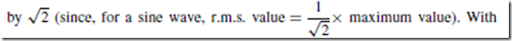total reflection, the standing-wave patterns of r.m.s. voltage and current consist of a succession of positive sine waves with the voltage node located at the current antinode and the current node located at the voltage antinode. The termination is a current nodal point. The r.m.s. values of current and voltage may be recorded on a suitable r.m.s. instrument moving along the line. Such measurements of the maximum and minimum voltage and current can provide a reasonably accurate indication of the wavelength, and also provide information regarding the amount of reflected energy relative to the incident energy that is absorbed at the termination, as shown below.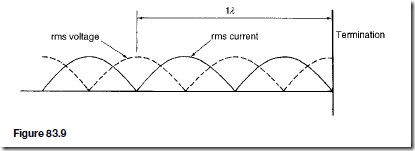Standing-wave ratio

Let the incident current flowing from the source of a mismatched low-loss transmission line be Ii and the current reflected at the termination be Ir . If IMAX is the sum of the incident and reflected current, and IMIN is their difference, then the standing-wave ratio (symbol s) on the line is defined as: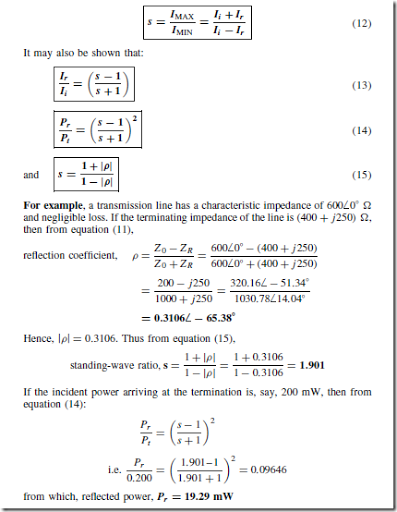# Attenuators

### Introduction

An attenuator is a device for introducing a specified loss between a signal source and a matched load without upsetting the impedance relationship necessary for matching. The loss introduced is constant irrespective of frequency; since reactive elements (L or C) vary with frequency, it follows that ideal attenuators are networks containing pure resistances. A fixed attenuator section is usually known as a ‘pad’.

Attenuation is a reduction in the magnitude of a voltage or current due to its transmission over a line or through an attenuator. Any degree of attenuation may be achieved with an attenuator by suitable choice of resistance values but the input and output impedances of the pad must be such that the impedance conditions existing in the circuit into which it is connected are not disturbed. Thus an attenuator must provide the correct input and output impedances as well as providing the required attenuation.

#### Two-port Networks

Networks in which electrical energy is fed in at one pair of terminals and taken out at a second pair of terminals are called two-port networks. Thus an attenuator is a two-port network, as are transmission lines, transformers and electronic amplifiers. If a network contains only passive circuit elements, such as in an attenuator, the network is said to be passive; if a network contains a source of e.m.f., such as in an electronic amplifier, the network is said to be active.

Figure 80.1(a) shows a T-network, which is termed symmetrical if ZA D ZB and Figure 80.1(b) shows a .r-network which is symmetrical if ZE= ZF. If ZA 6D ZB in Figure 80.1(a), and ZE 6D ZF in Figure 80.1(b), the sections are termed asymmetrical. Both networks shown have one common terminal, which may be earthed, and are therefore said to be unbalanced. The balanced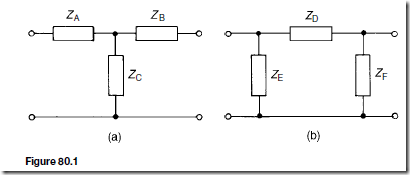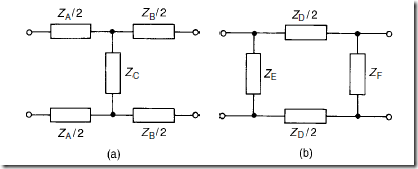form of the T-network is shown in Figure 80.2(a) and the balanced form of the .r-network is shown in Figure 80.2(b).

#### Characteristic Impedance

The input impedance of a network is the ratio of voltage to current (in complex form) at the input terminals. With a two-port network the input impedance often varies according to the load impedance across the output terminals. For any passive two-port network it is found that a particular value of load impedance can always be found which will produce an input impedance having the same value as the load impedance. This is called the iterative impedance for an asymmetrical network and its value depends on which pair of terminals is taken to be the input and which the output (there are thus two values of iterative impedance, one for each direction). For a symmetrical network there is only one value for the iterative impedance and this is called the characteristic impedance of the symmetrical two-port network.

#### Logarithmic Ratios

The ratio of two powers P1 and P2 may be expressed in logarithmic form as shown in chapter 50. Let P1 be the input power to a system and P2 the output power.

If logarithms to base 10 are used then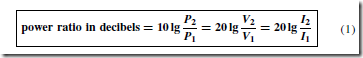For example, if, say, 5% of the power supplied to a cable appears at the output terminals then the attenuation in decibels is determined as follows:

From equation (1),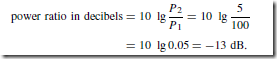Hence the attenuation (i.e. power loss) is 13 dB.

In another example, if an amplifier has a gain of 15 dB and the input power is 12 mW, the output power is determined as follows:

From equation (1),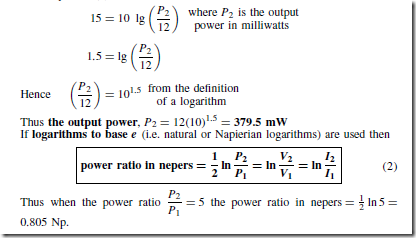The attenuation of filter sections and along a transmission line are of an exponential form and it is in such applications that the unit of the neper is used (see chapters 81 and 83).

Symmetrical T -attenuator

For the symmetrical T-pad attenuator is shown in Figure 80.3, the characteristic impedance R0 is given by: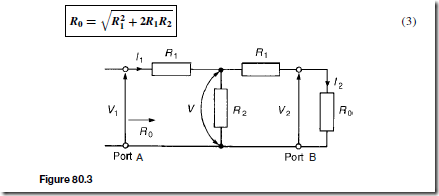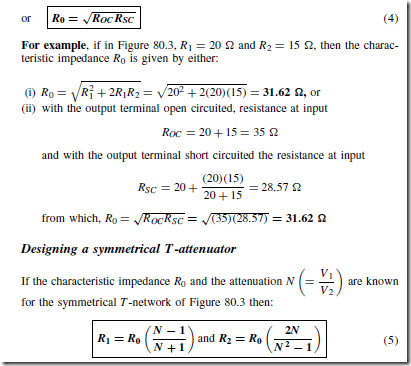For example, a T-section symmetrical attenuator pad to provide a voltage attenuation of 20 dB and having a characteristic impedance of 600 Q is designed as follows: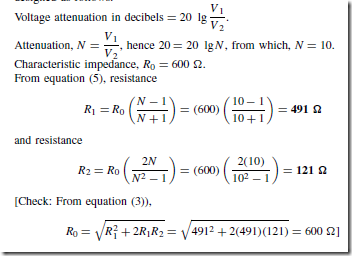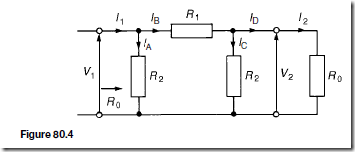Symmetrical p-attenuator

For the symmetrical .r-attenuator shown in Figure 80.4 the characteristic impedance R0 is given by: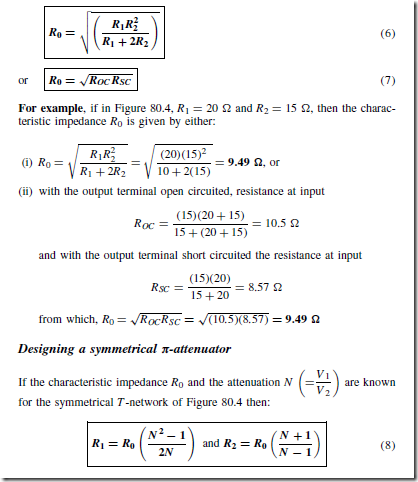For example, if a .r-section symmetrical attenuator is required to provide a voltage attenuation of 25 dB and have a characteristic impedance of 600 Q, then: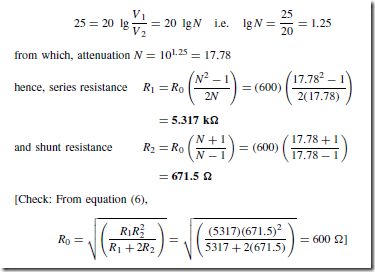Insertion Loss

Figure 80.5(a) shows a generator E connected directly to a load ZL . Let the current flowing be IL and the p.d. across the load VL . z is the internal impedance of the source.

Figure 80.5(b) shows a two-port network connected between the generator E and load ZL . The current through the load, shown as I2 , and the p.d. across the load, shown as V2 will generally be less than current IL and voltage VL of Figure 80.5(a), as a result of the insertion of the two-port network between generator and load.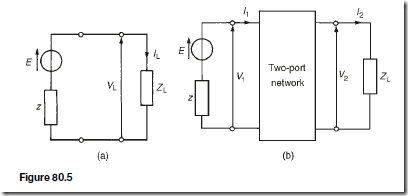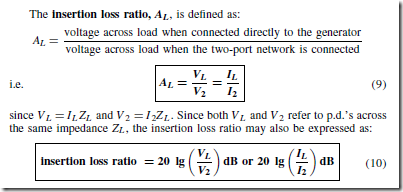When the two-port network is terminated in its characteristic impedance Z0 the network is said to be matched. In such circumstances the input impedance is also Z0, thus the insertion loss is simply the ratio of input to output voltage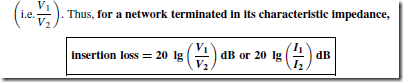For example, a 0– 3 kQ rheostat is connected across the output of a signal generator of internal resistance 500 Q. If a load of 2 kQ is connected across the rheostat, the insertion loss at a tapping of, say, 2 kQ is determined as follows:

The circuit diagram is shown in Figure 80.6. Without the rheostat in the ircuit the voltage across the 2 kQ load, VL (see Figure 80.7), is given by: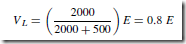With the 2 kQ tapping, the network of Figure 80.7 may be redrawn as shown in Figure 80.8, which in turn is simplified as shown in Figure 80.9. From Figure 80.9,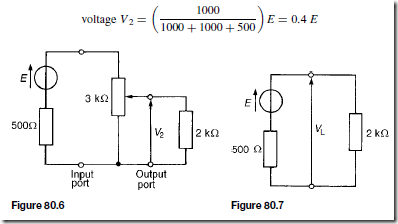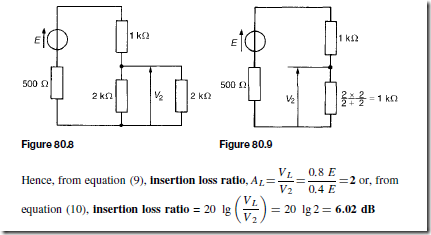Asymmetrical T - and p-sections

Figure 80.10(a) shows an asymmetrical T-pad section where resistance R1 6D 3. Figure 80.10(b) shows an asymmetrical .r-section where R2 6D R3.

When viewed from port A, in each of the sections, the output impedance is ROB; when viewed from port B, the input impedance is ROA. Since the sections are asymmetrical ROA does not have the same value as ROB.

Iterative impedance is the term used for the impedance measured at ne port of a two-port network when the other port is terminated with an impedance of the same value. For example, the impedance looking into port 1 of Figure 80.11(a) is, say, 500 Q when port 2 is terminated in 500 Q and the impedance looking into port 2 of Figure 80.11(b) is, say, 600 Q when port 1 is terminated in 600 Q. (In symmetric T- and .r-sections the two iterative impedances are equal, this value being the characteristic impedance of the section).

An image impedance is defined as the impedance which, when connected to the terminals of a network, equals the impedance presented to it at the opposite terminals. For example, the impedance looking into port 1 of Figure 80.12(a) is, say, 400 Q when port 2 is terminated in, say 750 Q, and the impedance seen looking into port 2 (Figure 80.12(b)) is 750 Q when port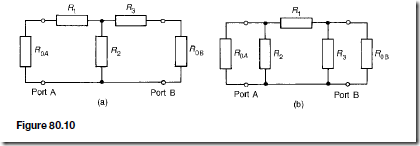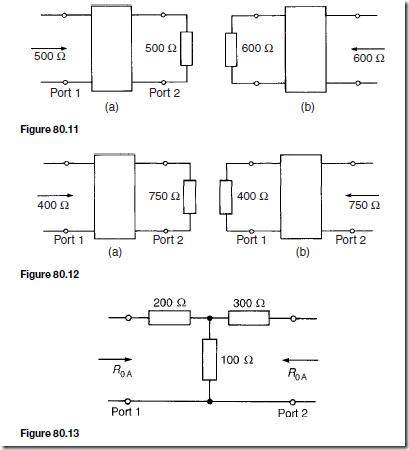1 is terminated in 400 Q. An asymmetrical network is correctly terminated when it is terminated in its image impedance. (If the image impedances are equal, the value is the characteristic impedance).

For example, an asymmetrical T-section attenuator is shown in Figure 80.13. The image and iterative impedances are determined as follows: The image impedance ROA seen at port 1 in Figure 80.13 is given by equation (4):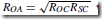where ROC and RSC refer to port 2 being respectively open-circuited and short circuited.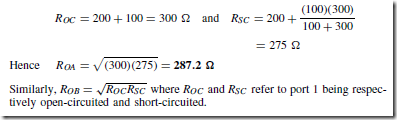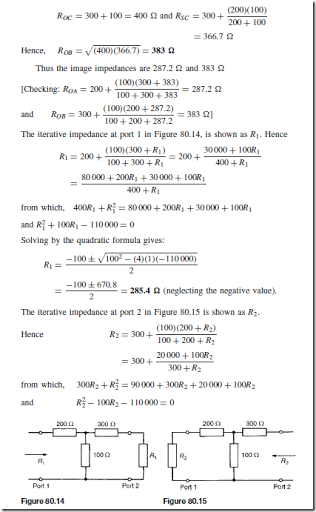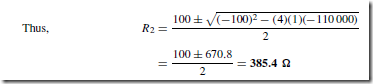Thus the iterative impedances of the section shown in Figure 80.13 are 285.4  and 385.4

The L-section Attenuator

For the L-section attenuator shown in Figure 80.16,